Test: Thermodynamics And Thermochemistry - 2

# Test: Thermodynamics And Thermochemistry - 2

Test Description

## 30 Questions MCQ Test Physical Chemistry | Test: Thermodynamics And Thermochemistry - 2

Test: Thermodynamics And Thermochemistry - 2 for Chemistry 2023 is part of Physical Chemistry preparation. The Test: Thermodynamics And Thermochemistry - 2 questions and answers have been prepared according to the Chemistry exam syllabus.The Test: Thermodynamics And Thermochemistry - 2 MCQs are made for Chemistry 2023 Exam. Find important definitions, questions, notes, meanings, examples, exercises, MCQs and online tests for Test: Thermodynamics And Thermochemistry - 2 below.
Solutions of Test: Thermodynamics And Thermochemistry - 2 questions in English are available as part of our Physical Chemistry for Chemistry & Test: Thermodynamics And Thermochemistry - 2 solutions in Hindi for Physical Chemistry course. Download more important topics, notes, lectures and mock test series for Chemistry Exam by signing up for free. Attempt Test: Thermodynamics And Thermochemistry - 2 | 30 questions in 90 minutes | Mock test for Chemistry preparation | Free important questions MCQ to study Physical Chemistry for Chemistry Exam | Download free PDF with solutions
 1 Crore+ students have signed up on EduRev. Have you?
Test: Thermodynamics And Thermochemistry - 2 - Question 1

### Enthalpy change for a reaction does not depend upon:

Test: Thermodynamics And Thermochemistry - 2 - Question 2

### The heat of combust ion of carbon to CO2 is –393 KJ/mol. The heat released upon format ion of 35.2 g of CO2 from carbon and oxygen gas is:

Test: Thermodynamics And Thermochemistry - 2 - Question 3

### Consider the reaction: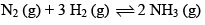Carried out at constant temperature and pressure. If ΔH and ΔU are enthalpy and internal energy changes for the reaction, which of the following expressions is true?

Detailed Solution for Test: Thermodynamics And Thermochemistry - 2 - Question 3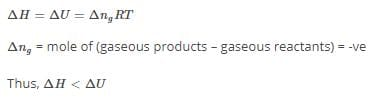Test: Thermodynamics And Thermochemistry - 2 - Question 4

The work done in ergs for the reversible expansion of one mole of an ideal gas from a volume of 10L to 20L is:

Detailed Solution for Test: Thermodynamics And Thermochemistry - 2 - Question 4

Final volume V2​=20L

initial volume V1​=10L

W = 2.303 nRTln(V2/V1​​)

=  1 x 298 x 10x 8.314 x 2.303 log2

Test: Thermodynamics And Thermochemistry - 2 - Question 5

Work done during isothermal expansion of 1 mole of an ideal gas from 10 atm to 1 atm at 300K is:

Test: Thermodynamics And Thermochemistry - 2 - Question 6

ΔE° of combustion of isobut ylene is x KJ mol-1. The value of ΔH° is:

Detailed Solution for Test: Thermodynamics And Thermochemistry - 2 - Question 6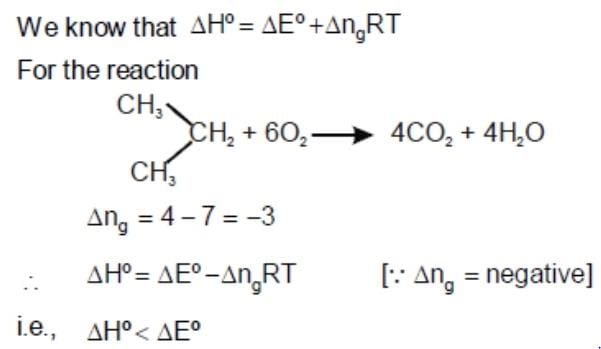Test: Thermodynamics And Thermochemistry - 2 - Question 7

The free energy change for a reversible reaction at equilibrium is:

Test: Thermodynamics And Thermochemistry - 2 - Question 8

The heat of neutralization of a strong acid and a strong alkali is 57.0 KJ mol–1. The heat released when 0.5 mole of HNO3 solution is mixed with 0.2 mole of KOH is:

Test: Thermodynamics And Thermochemistry - 2 - Question 9

Heat of formation of H2O is –286 KJ per mole and H2O2 is –188 KJ mol–1. The enthalpy change for the reaction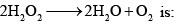Detailed Solution for Test: Thermodynamics And Thermochemistry - 2 - Question 9

ΔHreaction​=(∑Hproducts​)−(∑Hreactants​)

Δ Hreaction=[(ΔHO2​​)+(ΔHH2​O​)]−[ΔHH2​O2​​]

ΔHO2​​=0,ΔHH2​O​=−286KJ per mole, ΔHH2​O2​​=−188KJ per mole

put the values ,

ΔH{reaction}=-196 KJ per mole

Test: Thermodynamics And Thermochemistry - 2 - Question 10

The species which by definition has ZERO standard molar enthalpy of formation at 298 K is

Test: Thermodynamics And Thermochemistry - 2 - Question 11

Given that bond energies of H–H and Cl–Cl are 430 and 240 KJ mol–1 respectively, and ΔHf for HCl is -90 KJ mol-1. Bond enthalpy of HCl is:

Detailed Solution for Test: Thermodynamics And Thermochemistry - 2 - Question 11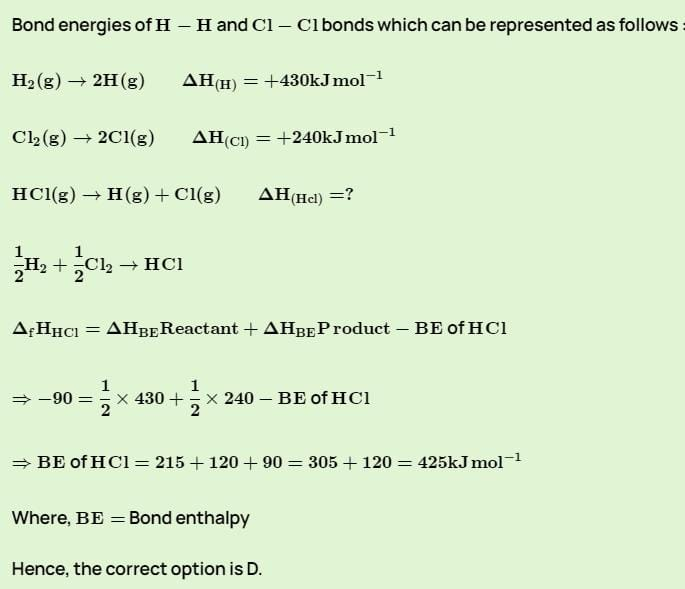Test: Thermodynamics And Thermochemistry - 2 - Question 12

Hess’s Law deals with:

Test: Thermodynamics And Thermochemistry - 2 - Question 13

One mole of methanol when burnt in O2 gives out 723 KJ mol–1 heat. If one mole of O2 is used, what will be the amount of heat observed:

Detailed Solution for Test: Thermodynamics And Thermochemistry - 2 - Question 13

(1) CH3OH +3232O2→→CO2 + 2H2O, ΔH∆H=-723 kJ

The heat evolved for one mole of oxygen = -723x2/3 = -482 kJ

Test: Thermodynamics And Thermochemistry - 2 - Question 14

The enthalpy and entropy change for the reaction: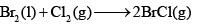Are 30 KJ mol–1 and 105 JK–1mol–1 respect ively. The temperature at which the reaction will be in equilibrium is:

Test: Thermodynamics And Thermochemistry - 2 - Question 15

The change in the entropy for the fusion of 1 mole of ice is:

(Melting point of ice = 273 K, molar enthalpy of fusion of ice = 6.0 KJ mol–1):

Detailed Solution for Test: Thermodynamics And Thermochemistry - 2 - Question 15

Change in Entropy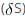=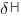(Enthaply of fusion) /T (Temperature)

= 60*10/ 273

= 21.97 J/K/mol

Test: Thermodynamics And Thermochemistry - 2 - Question 16

For a spontaneous process, the correct statement is:

Test: Thermodynamics And Thermochemistry - 2 - Question 17

The abso lute enthalpy of neutralization of the reaction: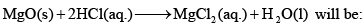Test: Thermodynamics And Thermochemistry - 2 - Question 18

For the reaction of one mole of zinc dust with one mole of sulphuric acid in a bomb calorimeter, ΔU and W corresponds to:

Test: Thermodynamics And Thermochemistry - 2 - Question 19

Standard enthalpy and standard entropy changes for the oxidation of NH3 at 298 K are –382.64 KJ mol–1 and – 145.6 JK–1mol–1, respectively. Standard Gibb’s energy change for the same reaction at 298 K is:

Test: Thermodynamics And Thermochemistry - 2 - Question 20

ΔG = ΔH-TΔS was given by:

Test: Thermodynamics And Thermochemistry - 2 - Question 21

The heat capacity of 10 mol of an ideal gas at a certain temperature is 300 JK–1 at constant pressure. The heat capacity of the same gas at the same temperature and at constant volume would be:

Test: Thermodynamics And Thermochemistry - 2 - Question 22

The internal pressure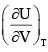of a real gas is related to the compressibility factor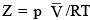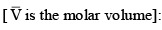Test: Thermodynamics And Thermochemistry - 2 - Question 23

Entropy is:

(I) It is the degree of disorder of randomness in a system
(II) Entropy is a state function and mixing is always positive
(III) Entropy is a intensive property
(IV) Entropy of solid is minimum and gases is maximum
(V) Entropy increase wit h temperature, complexity and atomicity

The correct statement is:

Test: Thermodynamics And Thermochemistry - 2 - Question 24

From the following pairs, choose the substances with the higher entropy per mole at a given temperature:

(I) O2 gas at 5 atm or O2 gas at 0.5 atm
(II) Br2 (L) or Br2 (g)

Test: Thermodynamics And Thermochemistry - 2 - Question 25

For the gaseous substance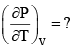Test: Thermodynamics And Thermochemistry - 2 - Question 26

Among the following, the system require higher amount of thermal energy to giving the temperature 800C:

Test: Thermodynamics And Thermochemistry - 2 - Question 27

What is the internal pressure for 4 mole of Vander Waals gas:

Test: Thermodynamics And Thermochemistry - 2 - Question 28

Which of the following statement is correct: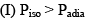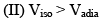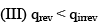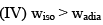Detailed Solution for Test: Thermodynamics And Thermochemistry - 2 - Question 28

Explanation : The magnitude of the work for the isothermal process for both expansion and compression is greater than the magnitude of the work for the adiabatic process.

As we know that PV = constant

The work done by the gas is equal to the area under the relevant pressure -volume isotherm.

Test: Thermodynamics And Thermochemistry - 2 - Question 29

A piston-cylinder contains 0.5 kg of air at 500 kPa and 500 K. The air expands in a process so the pressure is linearly decreasing with volume to a final state of 100 kPa and 300 K. Find the work in the process.

Detailed Solution for Test: Thermodynamics And Thermochemistry - 2 - Question 29

Ans: d

Explanation: Work = ⌠ PdV = (1 / 2)(P1 + P2)(V2 – V1)

V1 = mR, T1 / P1 = 0.5 × 0.287 × (500 / 500) = 0.1435 m3

V2 = mR, T2 / P2 = 0.5 × 0.287 × (300 / 100) = 0.4305 m3

W = (1 / 2)(500 + 100)(0.4305 – 0.1435) = 86.1 kJ.

Test: Thermodynamics And Thermochemistry - 2 - Question 30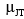for ideal and Vander Waals gases respectively:

## Physical Chemistry

85 videos|131 docs|67 tests
 Use Code STAYHOME200 and get INR 200 additional OFF Use Coupon Code
Information about Test: Thermodynamics And Thermochemistry - 2 Page
In this test you can find the Exam questions for Test: Thermodynamics And Thermochemistry - 2 solved & explained in the simplest way possible. Besides giving Questions and answers for Test: Thermodynamics And Thermochemistry - 2, EduRev gives you an ample number of Online tests for practice

## Physical Chemistry

85 videos|131 docs|67 tests